# Math Worksheets Printable

📆 25 Jun 2022
🔖 Math Category
📂 Gallery Type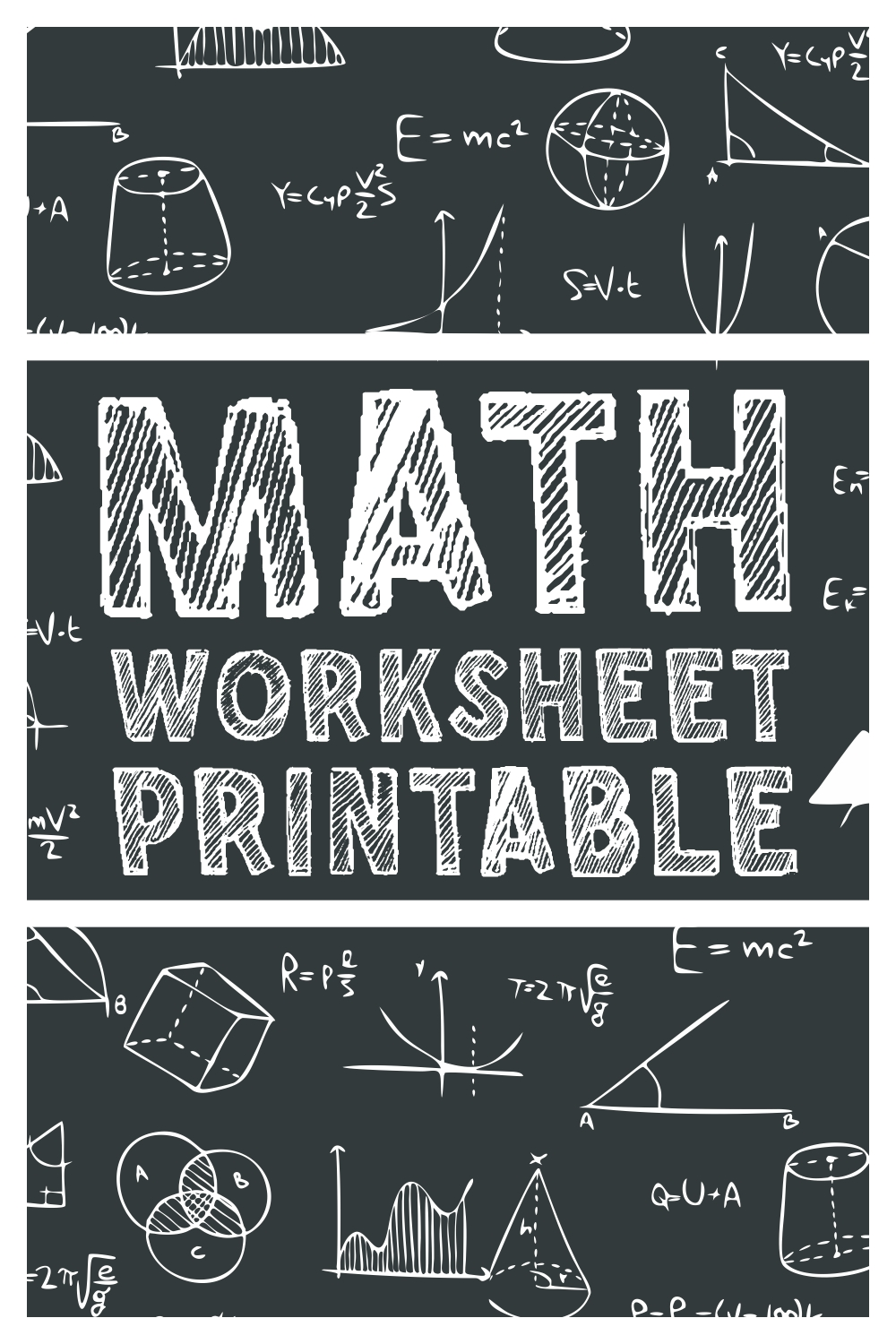10 Images of Math Worksheets Printable

Addition, Substraction,Division, and Multiple, we have all in here. See the complete Math Worksheets Printable here!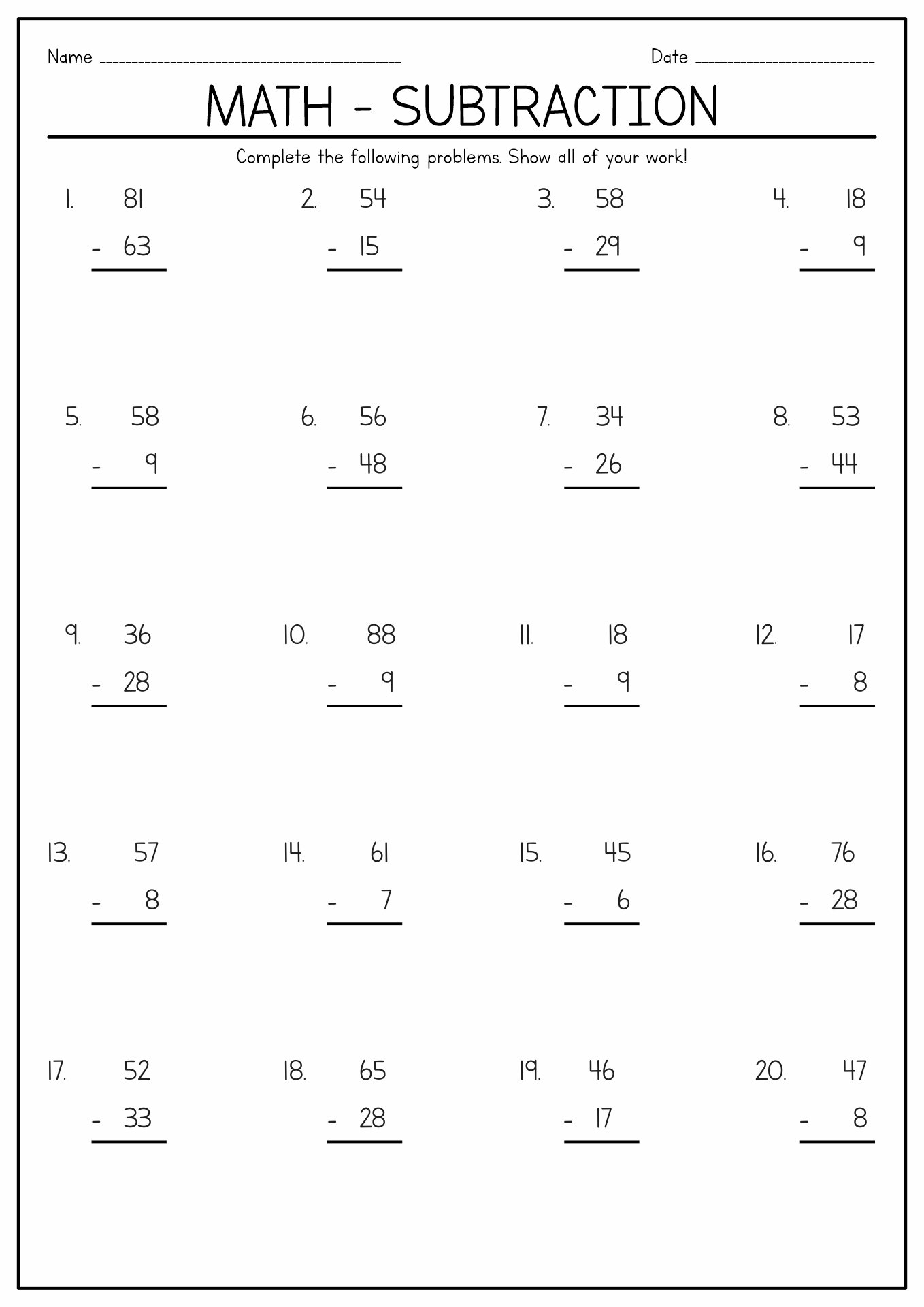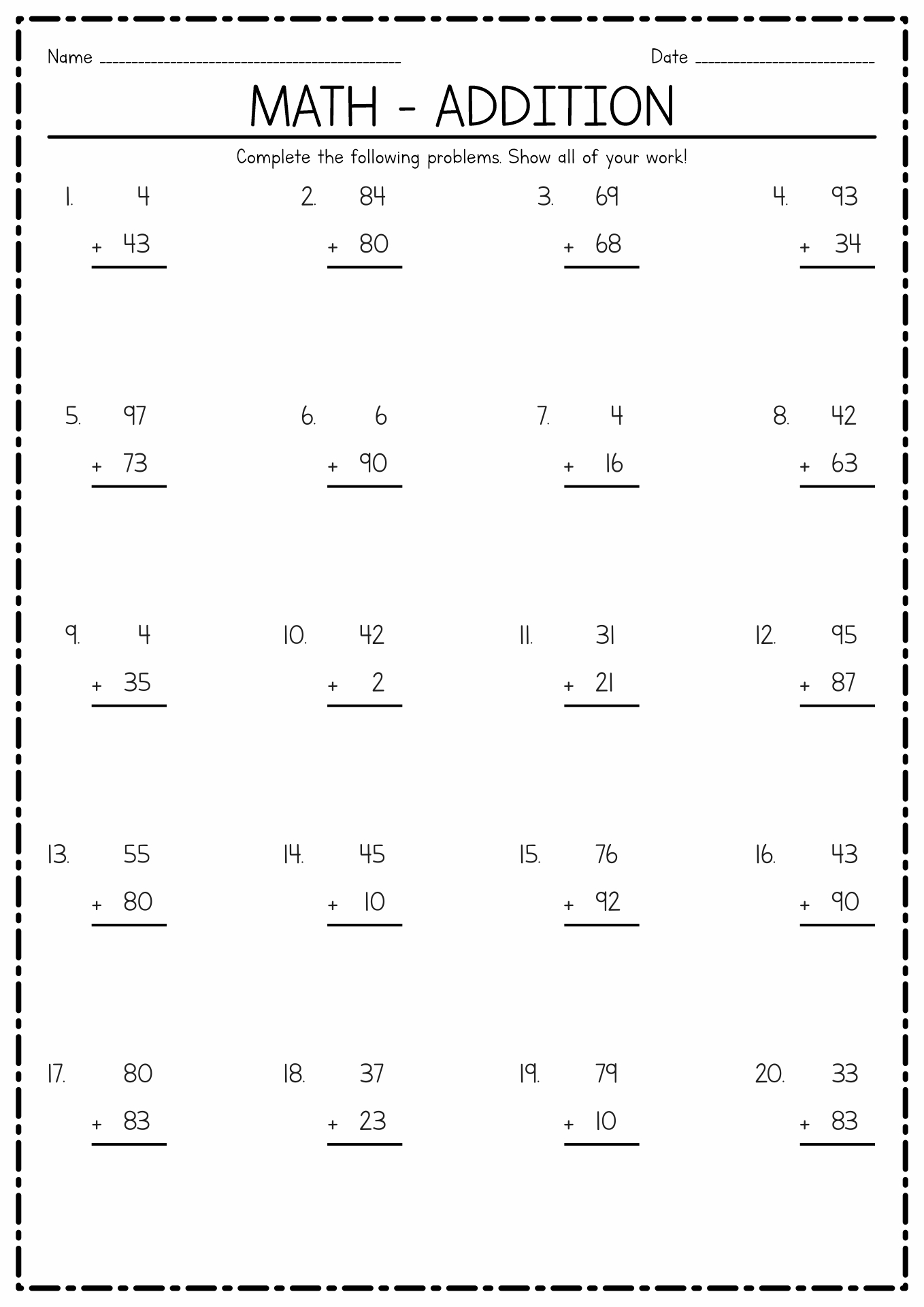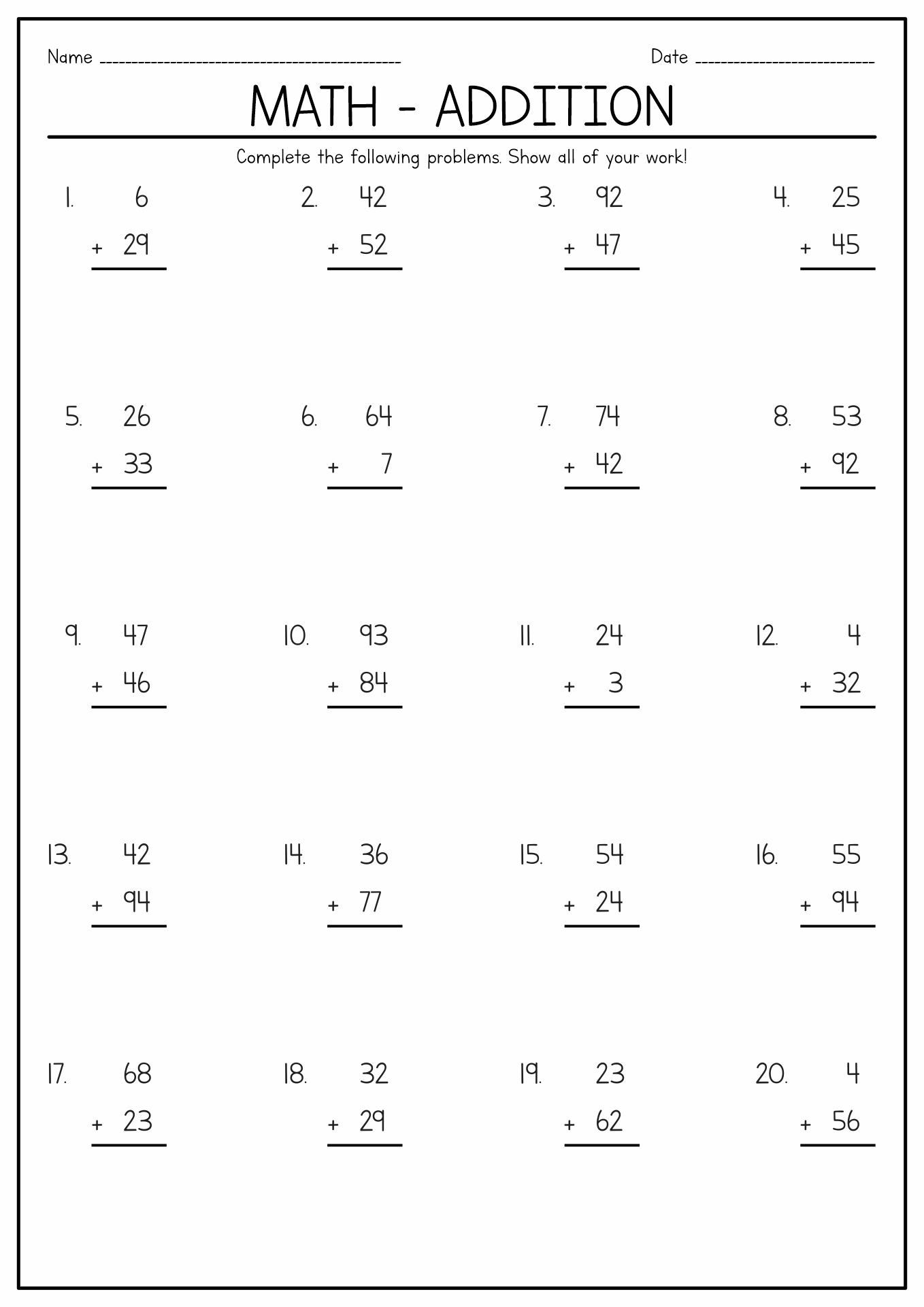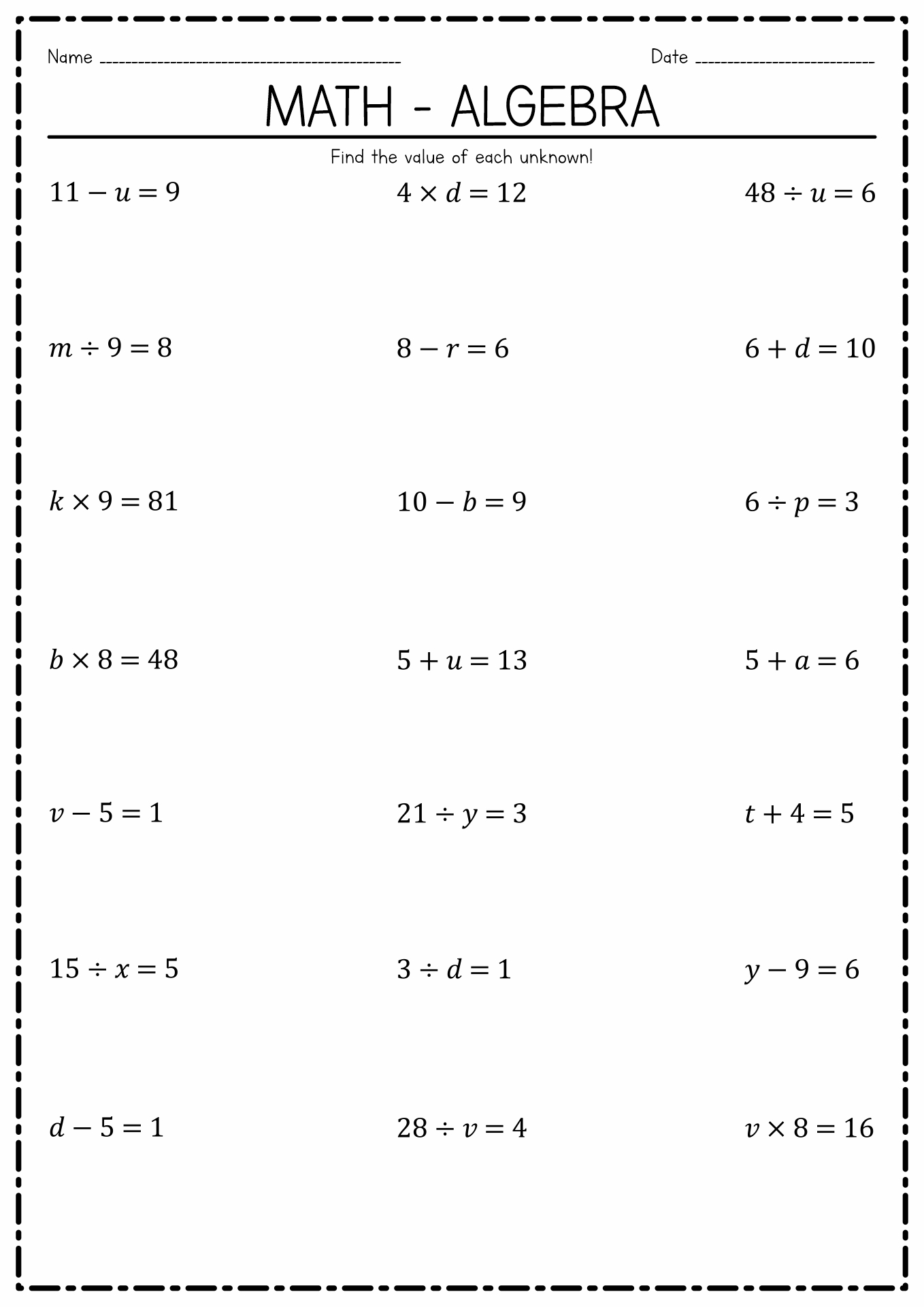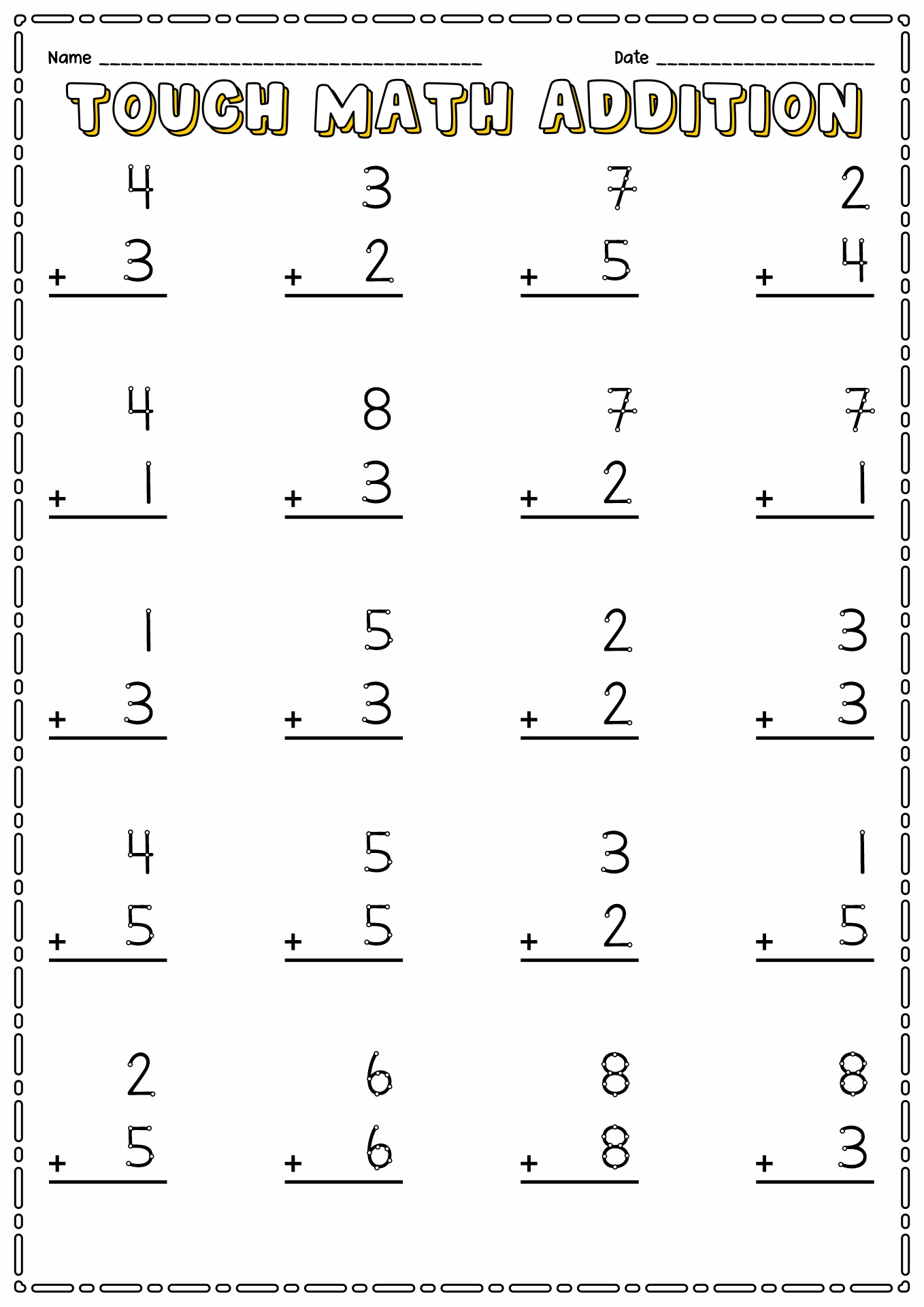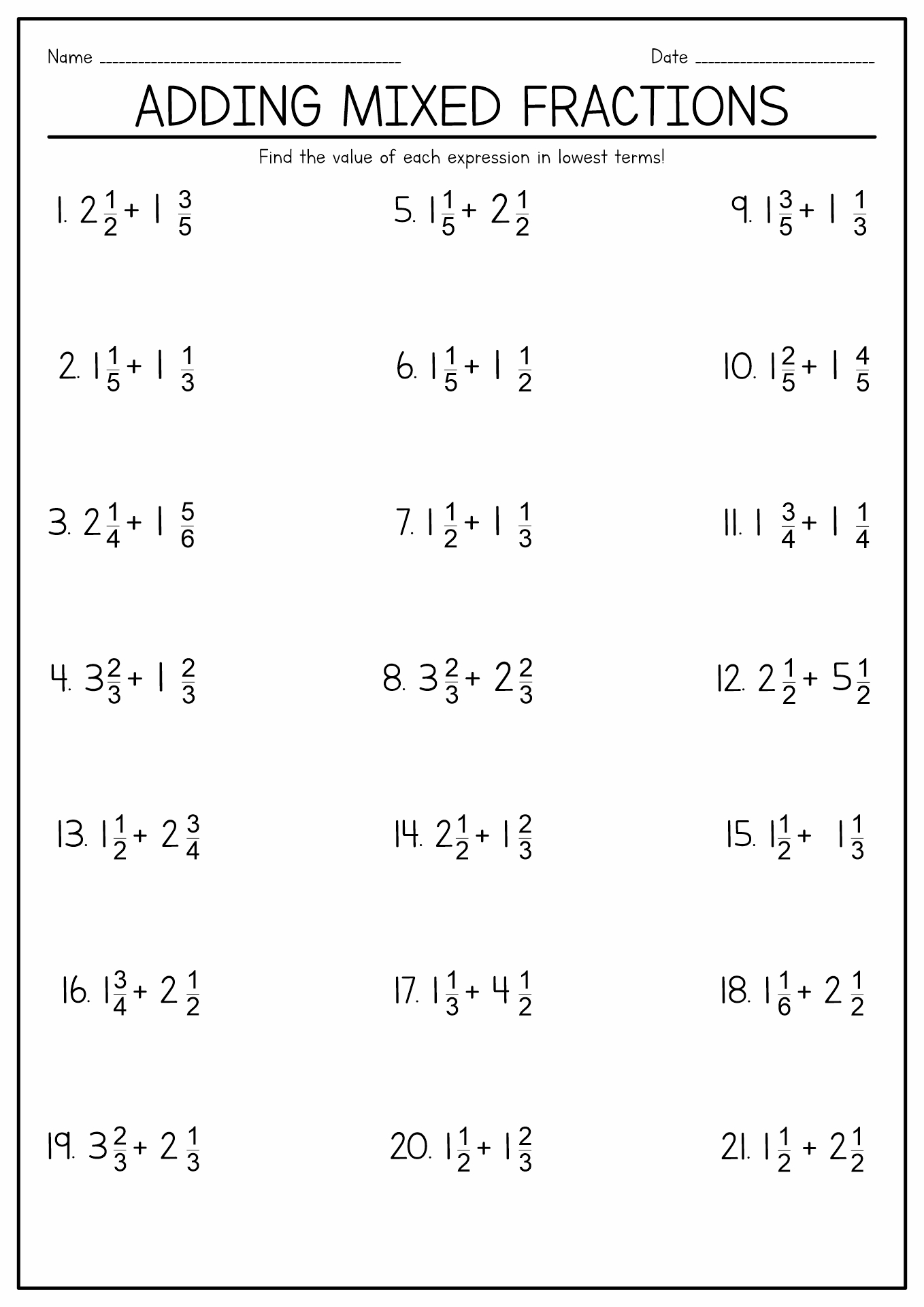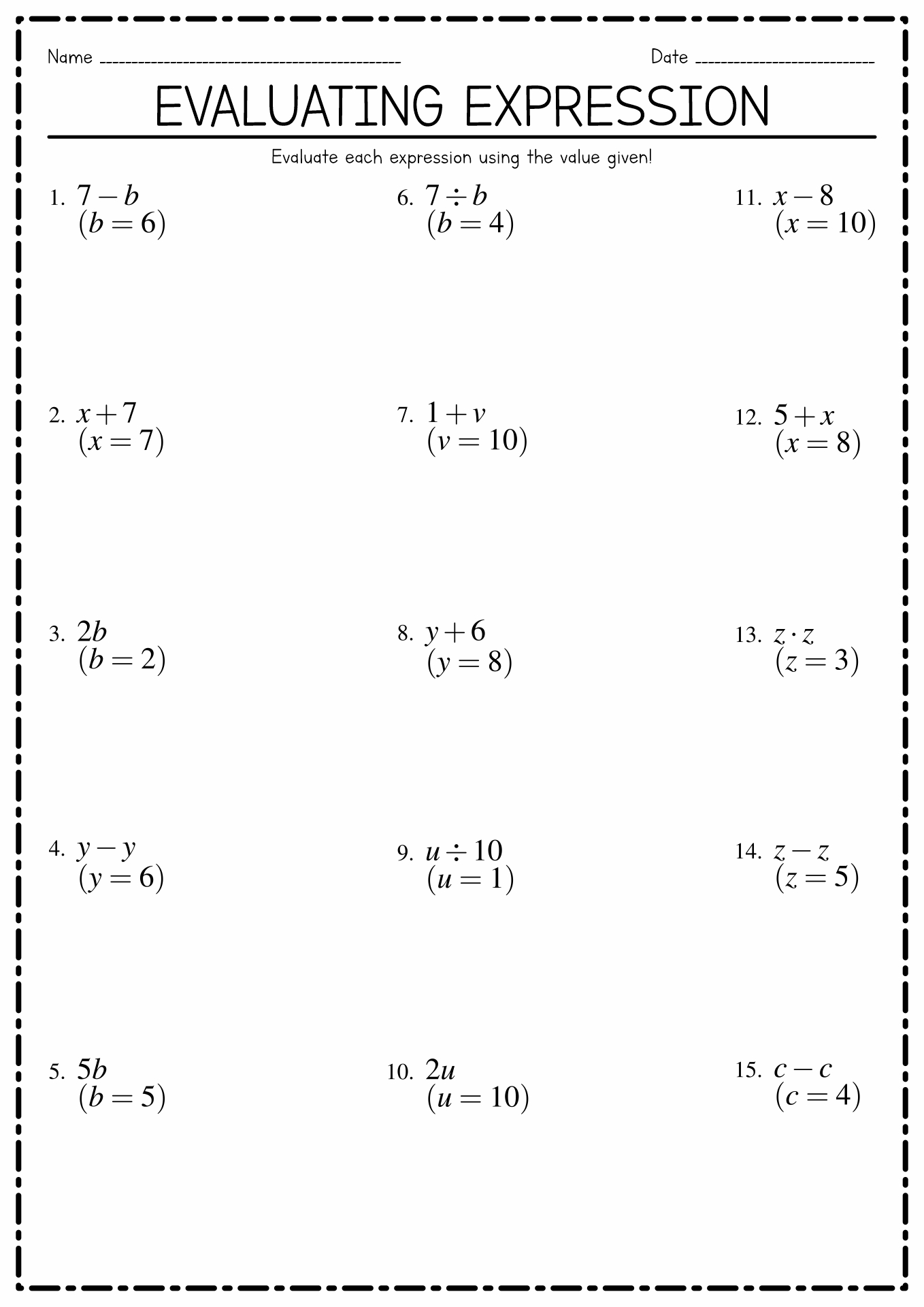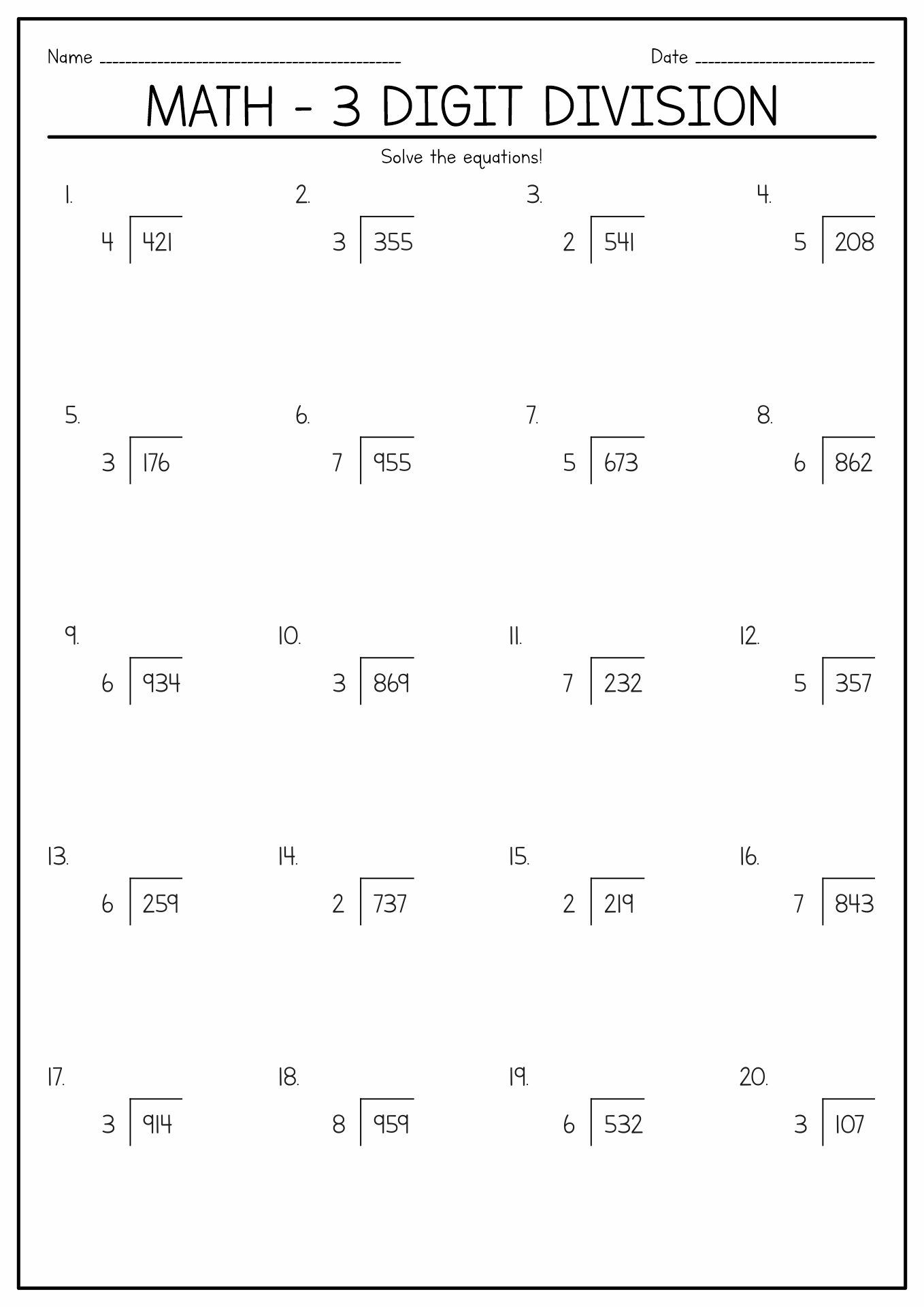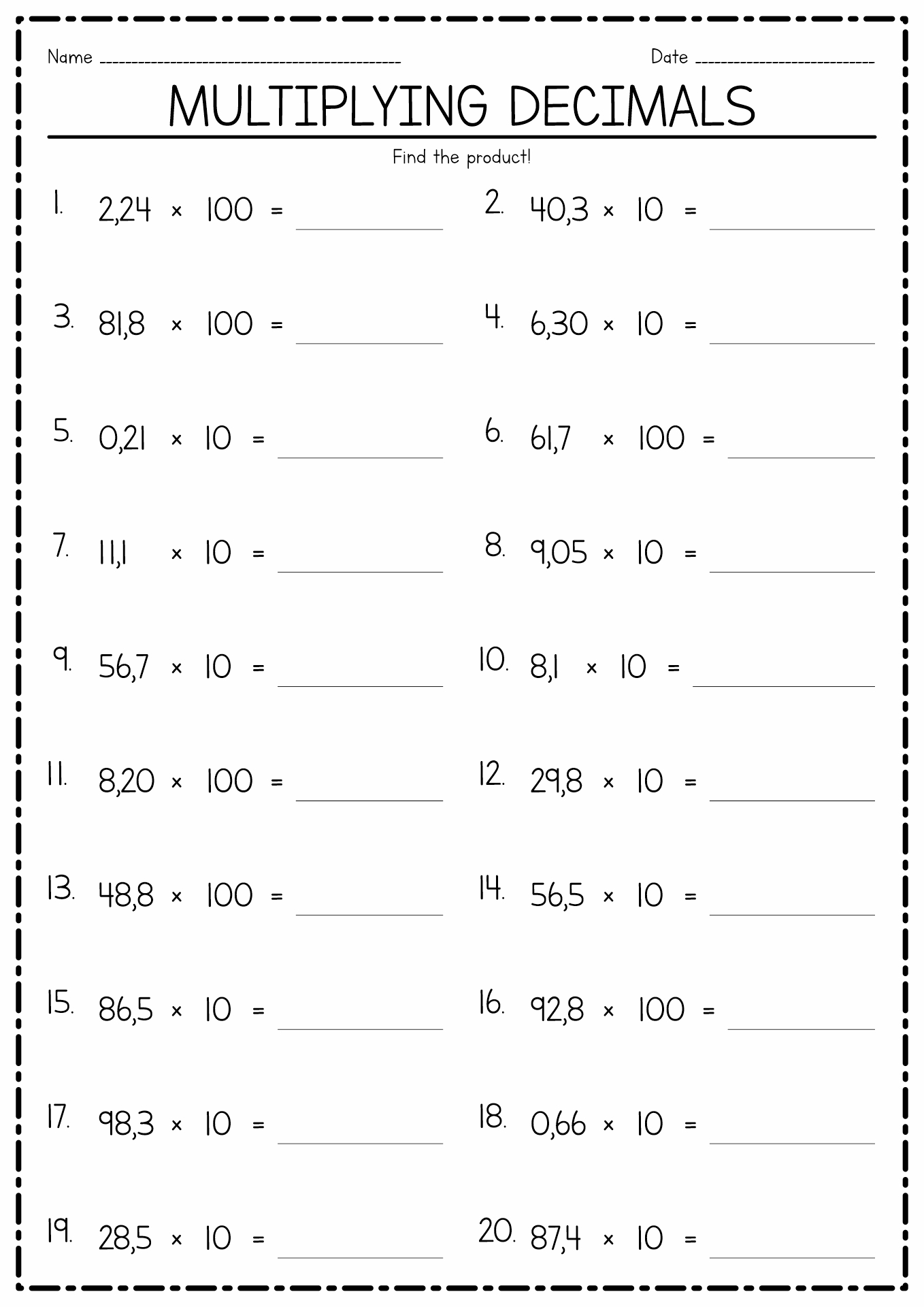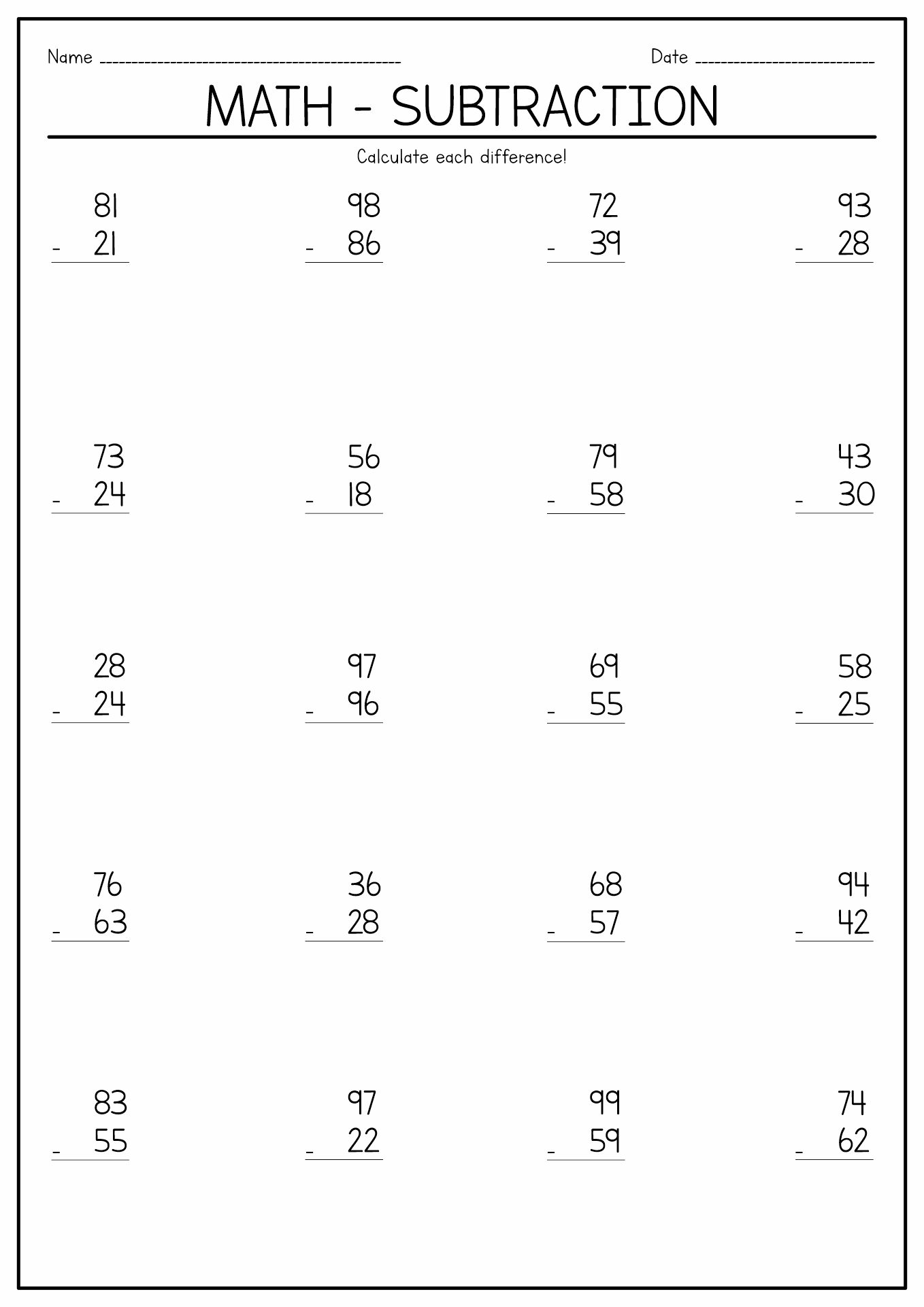Know more about math through these Math Worksheets Printable!

Summary: Mathematical ability is the competency to solve various quantitative problems. It includes mathematical word, computational and number-concept problems. Mathematical reasoning, number facility, deductive reasoning, inductive reasoning, and information ordering are essential mathematical abilities to master. Mathematics plays an essential role in diverse elements of human life. It provides a solid foundation for understanding and engaging with the world.

### What is the Definition of Math Ability?

Everyone must be familiar with mathematics. It is one of the essential knowledge because math has many vital roles in human everyday life. Try to think through your day and count how many you encountered math-related activities or objects. Do you realize? You did a count, and that is one of the math-related activities. According to the American Psychology Association, mathematical ability is the competency to solve various quantitative problems. It includes mathematical word, computational and number-concept problems. It also means the ability to follow instructions and do calculations with mechanical tools. There are some mathematical abilities that people should master to help them in their daily life. Mathematical reasoning (the ability to pick the correct method to solve math problems), number facility (the capability to do addition, subtraction, division, and multiplication), deductive reasoning (the ability to use general math rules to give a logical answer), inductive reasoning (the capability to connect pieces of information to produce a conclusion), and information ordering (the ability to put things and moments in logical order) are essential mathematical abilities to master.

### How Many Branches of Mathematics Are There?

Mathematics is a complicated knowledge with many branches of topics. Each of the topic branches relates to and completes each other. Below are some branches of mathematics that students should master:

• Arithmetic: This branch of mathematics deals with numbers and the four basic operations (addition, subtraction, division, and multiplication).
• Algebra: This branch of mathematics discusses unknown quantities and methods to discover them. In algebra, an English alphabet is used to represent the unknown numbers.
• Geometry: This branch talks about the shapes and sizes of figures and their properties. The point, line, angle, surface, and solid entities are geometry's essential elements of geometry.
• Trigonometry: This branch of mathematics deals with the connection between angles and sides of the triangle.
• Analysis: Mathematical analysis is the branch of mathematics that deals with extended functions, limits, and related theories. Differentiation, integration, measure, infinite sequences, series, and analytical functions are examples of mathematical analysis topics.

### Why is Mathematic One of the Essential Knowledge for Humans?

Mathematics plays an essential role in diverse elements of human life. It provides a solid foundation for understanding and engaging with the world. It is essential for functional applications in many fields, such as engineering, technology, and finance. Without mathematics, humans cannot create the tools to plan buildings and bridges, develop advanced computer algorithms, or manage individual and business finances effectively. Mathematical principles and calculations create the base in these areas, enabling us to decipher complex problems, make precise predictions, and optimize procedures.

Learning mathematics also trains our brains and minds to think logically, analyze situations carefully, and devise strategies to solve complicated problems. This analytical mindset exceeds the realm of mathematics and saturates other knowledge disciplines and real-life moments. Developing mathematical reasoning helps us create educated decisions, estimate upcoming risks, and approach challenges systematically. It enhances our capability to steer the complexities of the world and provides us with valuable cognitive tools. Some researchers also stated that learning mathematics helps people to develop their abstract thinking and creativity. It also provides a universal language to explain patterns, relationships, and systems. Mathematics enables us to explore the realms of abstract ideas and theoretical concepts and to turn the concept into something actual.

### How to Introduce Mathematic to Young Students?

Mathematics is essential knowledge to learn for students. It will help them to build numeral knowledge. Parents can start teaching their kids about mathematics before they begin formal education at school. Some experts stated that between the ages 2-8 years old is the golden moment for kids to develop math skills. It is essential to help the children discover the mathematical world in fun and exciting ways. It will make them less nervous and not feel pressured while learning. Parents or teachers can start math learning through fun activities such as knowing and understanding various shapes, counting using toys or daily objects, playing many games or puzzles, watching movies or videos with mathematic themes, singing math songs, and playing the compare and contrast games. It is also essential for parents and teachers to support the children while learning.

### Who Invented Math?

Mathematics is a natural old concept of knowledge. Hence, it is tough to credit the original inventors of mathematics. However, many mathematicians crowned the Greeks as the developer of the modern mathematics we learn nowadays. Some people also give credit to Archimedes as the father of mathematics. The term "mathematic" itself came from the Greek word "mathema", which means "knowledge." Many mathematicians agree that the four basic operations (addition, subtraction, division, and multiplication) are the foundation of numeral knowledge, as it has been discovered more than 4.000 years ago.

The information, names, images and video detail mentioned are the property of their respective owners & source.

### Popular Categories

Have something to tell us about the gallery?

Submit